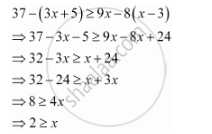CBSE (Arts) Class 11CBSE
Share

# Solve the Given Inequality for Real X: 37 ­– (3x + 5) ≥ 9x – 8(X – 3) - CBSE (Arts) Class 11 - Mathematics

ConceptAlgebraic Solutions of Linear Inequalities in One Variable and Their Graphical Representation

#### Question

Solve the given inequality for real x: 37 ­– (3x + 5) ≥ 9x – 8(– 3)

#### SolutionThus, all real numbers x,which are less than or equal to 2, are the solutions of the given inequality.

Hence, the solution set of the given inequality is (–∞, 2].

Is there an error in this question or solution?

#### APPEARS IN

NCERT Solution for Mathematics Textbook for Class 11 (2018 to Current)
Chapter 6: Linear Inequalities
Q: 14 | Page no. 122
Solution Solve the Given Inequality for Real X: 37 ­– (3x + 5) ≥ 9x – 8(X – 3) Concept: Algebraic Solutions of Linear Inequalities in One Variable and Their Graphical Representation.
S